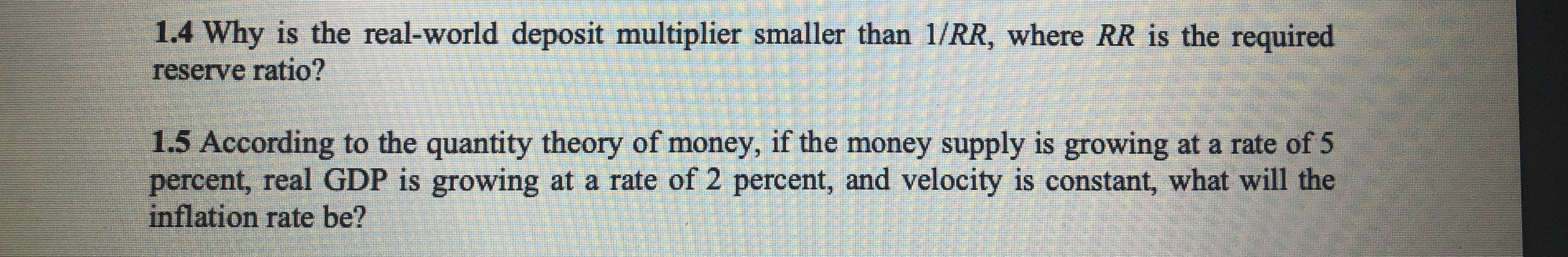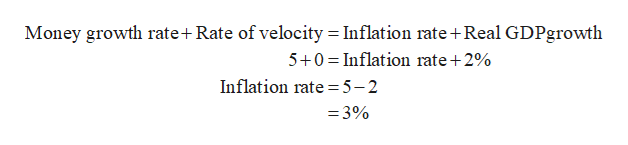# 1.4 Why is the real-world deposit multiplier smaller than 1/RR, where RR is the requiredreserve ratio?1.5 According to the quantity theory of money, if the money supply is growing at a rate of 5percent, real GDP is growing at a rate of 2 percent, and velocity is constant, what will theinflation rate be?

Question
2 views

how do i answer 1.5 . it seems like something is missing for me to do the equationhelp_outlineImage Transcriptionclose1.4 Why is the real-world deposit multiplier smaller than 1/RR, where RR is the required reserve ratio? 1.5 According to the quantity theory of money, if the money supply is growing at a rate of 5 percent, real GDP is growing at a rate of 2 percent, and velocity is constant, what will the inflation rate be? fullscreen
check_circle

Step 1

Quantity theory of money refers to the relationship between price level and money supply. This relation can be numerically expressed as MV = PT.

Step 2

According to quantity theory of money, MV = PT (Money growth + velocity = inflation rate + ...help_outlineImage TranscriptioncloseMoney growth rate+ Rate of velocity = Inflation rate + Real GDPgrowth 5+0 Inflation rate +2% Inflation rate =5-2 -3% fullscreen

### Want to see the full answer?

See Solution

#### Want to see this answer and more?

Solutions are written by subject experts who are available 24/7. Questions are typically answered within 1 hour.*

See Solution
*Response times may vary by subject and question.
Tagged in